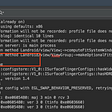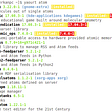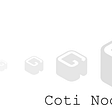# Intro to Web Scraping

`from bokeh.resources import CDNfrom bokeh.embed import file_htmlhtml = file_html(p, CDN, "NZ_City_Letter_Analysis")from IPython.core.display import HTMLHTML(html)`

# What is Web Scraping

`import scrapyclass BlogSpider(scrapy.Spider):    name = 'blogspider'    start_urls = ['https://blog.scrapinghub.com']    def parse(self, response):        for title in response.css('.post-header>h2'):            yield {'title': title.css('a ::text').get()}        for next_page in response.css('a.next-posts-link'):            yield response.follow(next_page, self.parse)`
`class NameSpider(scrapy.Spider):    name = 'names'    start_urls = ['https://nzhistory.govt.nz/culture/maori-language-week/1000-maori-place-names/']    def parse(self,response):        def extract_from_table(table_row,table_col):            return response.xpath(f"//tr[{table_row}]//td[{table_col}]//text()").get()        for i in range(2,1000):            yield {                'Place Name' : extract_from_table(i,1),                'Components' : extract_from_table(i,2),                'Meaning' : extract_from_table(i,3)            }`
`import pandas as pdimport collectionsfrom collections import OrderedDictimport operatorimport matplotlib.pyplot as pltimport numpy as npimport mathfrom bokeh.io import show, output_filefrom bokeh.plotting import figurefrom bokeh.models import ColumnDataSourcefrom bokeh.models.tools import HoverToolnames_df = pd.read_csv('names.csv', header=0, sep=',', quotechar='"')nz_names = names_df['Place Name'].tolist()nz_dict = { i : 0 for i in nz_names }letters = 'ABCDEFGHIJKLMNOPQRSTUVWXYZ'lcount = dict(OrderedDict([(l, 0) for l in letters]))for name in nz_names:    nz_dict[name] = dict(OrderedDict([(l, 0) for l in letters]))    city_dict = nz_dict[name]    for c in name:        if c.upper() in letters:            city_dict[c.upper()] += 1total_df = pd.DataFrame.from_dict(nz_dict)total_df = total_df.Tmax_letters_cities = total_df.idxmax().tolist()lettercounts = total_df.loc[total_df.idxmax()].max().tolist()maxletters = dict(OrderedDict([(l, 0) for l in letters]))for i,l in enumerate(letters):    maxletters[l] = max_letters_cities[i]    maxletters[l] = (lettercounts[i])summary_df = pd.DataFrame()scale = 1summary_df['Word_Name'] = total_df.idxmax()summary_df['Count'] = total_df.loc[total_df.idxmax()].max()source = ColumnDataSource(summary_df)output_file("letter_count.html")hover = HoverTool()hover.tooltips=[    ('Word', '@Word')]p = figure(x_range=summary_df.index.tolist(), plot_height=250, title="Letter Counts",           toolbar_location=None)p.vbar(x='index', top='Count', width=0.9,source=source)p.add_tools(hover)p.xgrid.grid_line_color = Nonep.y_range.start = 0show(p)`

--

--

--

## More from Jack McKew

Engineer | Software Developer | Data Scientist

Love podcasts or audiobooks? Learn on the go with our new app.

## 4 Powerful Reasons That Spotlight Why Michelle Obama Is a Genuine Leader## How to better use logs on Android## Install Software On Arch Linux OS## Top 8 Decisive Questions You Need to Ask Software Vendors## Sinatra — Movies catalog (Part-1)## Coti Node Hardening Guide — Part 3## Jack McKew

Engineer | Software Developer | Data Scientist

## Masai Collaboration project-Tata-Cliq website clone## How Much Does It Cost to Develop a TaskRabbit Clone App?## Streamflow — Money Streams Money Flows